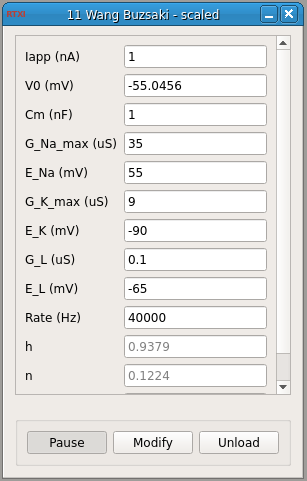### Wang-Buzsaki Model Neuron

Requirements: None
Limitations: NoneThe Wang-Buzsaki model uses the Hodgkin-Huxley formalism to describe a single-compartment neuron with sodium and potassium conductances. For the transient sodium current, the activation variable m is assumed fast and substituted by its steady-state function.

Wang XJ, Buzsáki G (1996) Gamma oscillation by synaptic inhibition in a hippocampal interneuronal network model. J. Neurosci. 16: 6402–6413.

#### Input Channels

1. input(0) - Istim : input current (A)

#### Output Channels

1. output(0) - Vm : membrane voltage (V)

#### Parameters

1. Iapp - applied current (nA)
2. V0 - voltage (mV)
3. Cm - membrane capacitance (nF/cm^2)
4. G_Na_max - max. Na+ conductance density (uS/cm^2)
5. E_Na - Na+ reversal potential (mV)
6. G_K_max - max. K+ conductance density (uS/cm^2)
7. E_K - K+ reversal potential (mV)
8. G_L - leak channel conductance density (uS/cm^2)
9. E_L - leak channel reversal potential (mV)
10. rate - rate of integration (Hz)

#### States

1. h - sodium inactivation
2. n - potassium inactivation
3. Time - time (s)